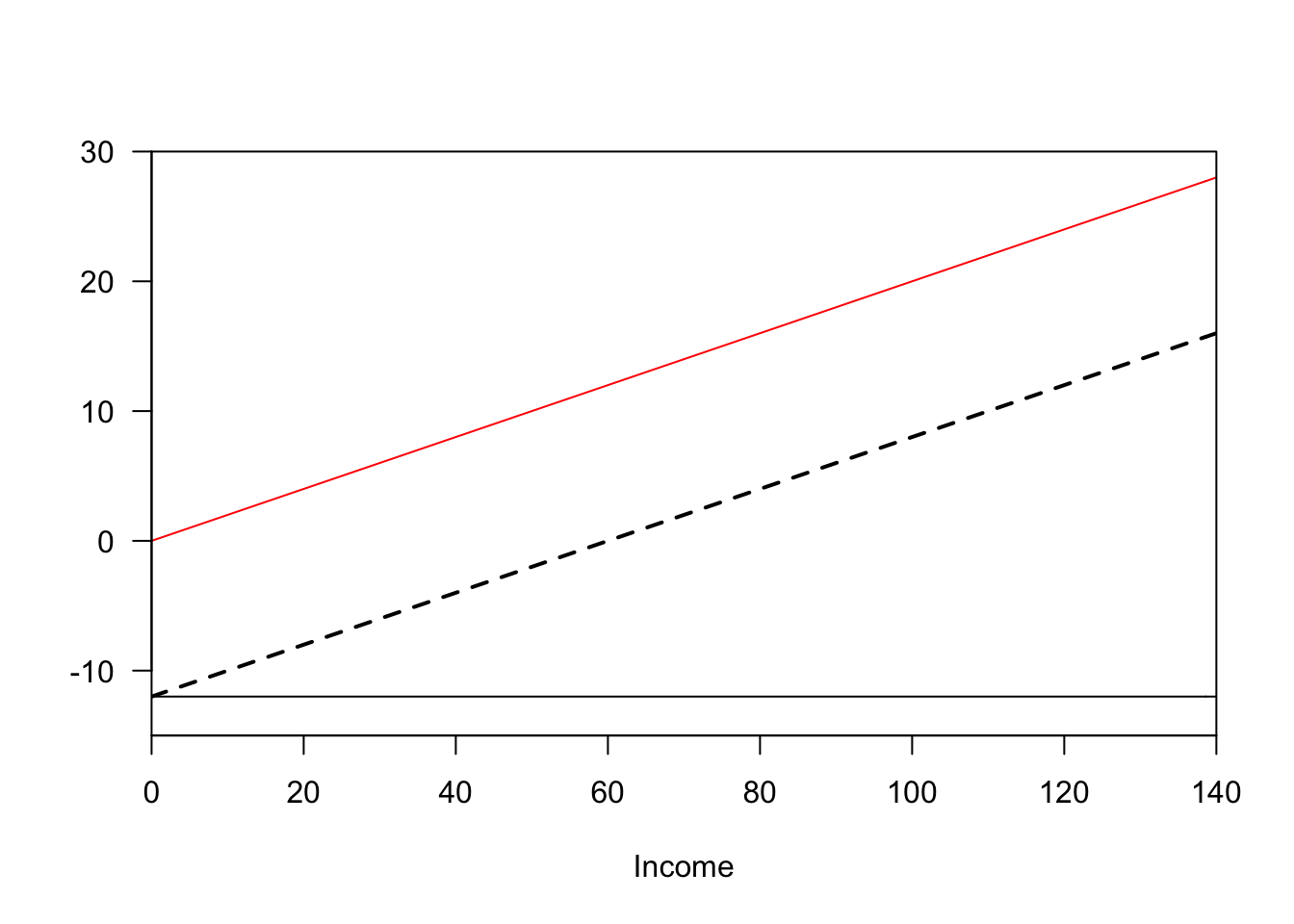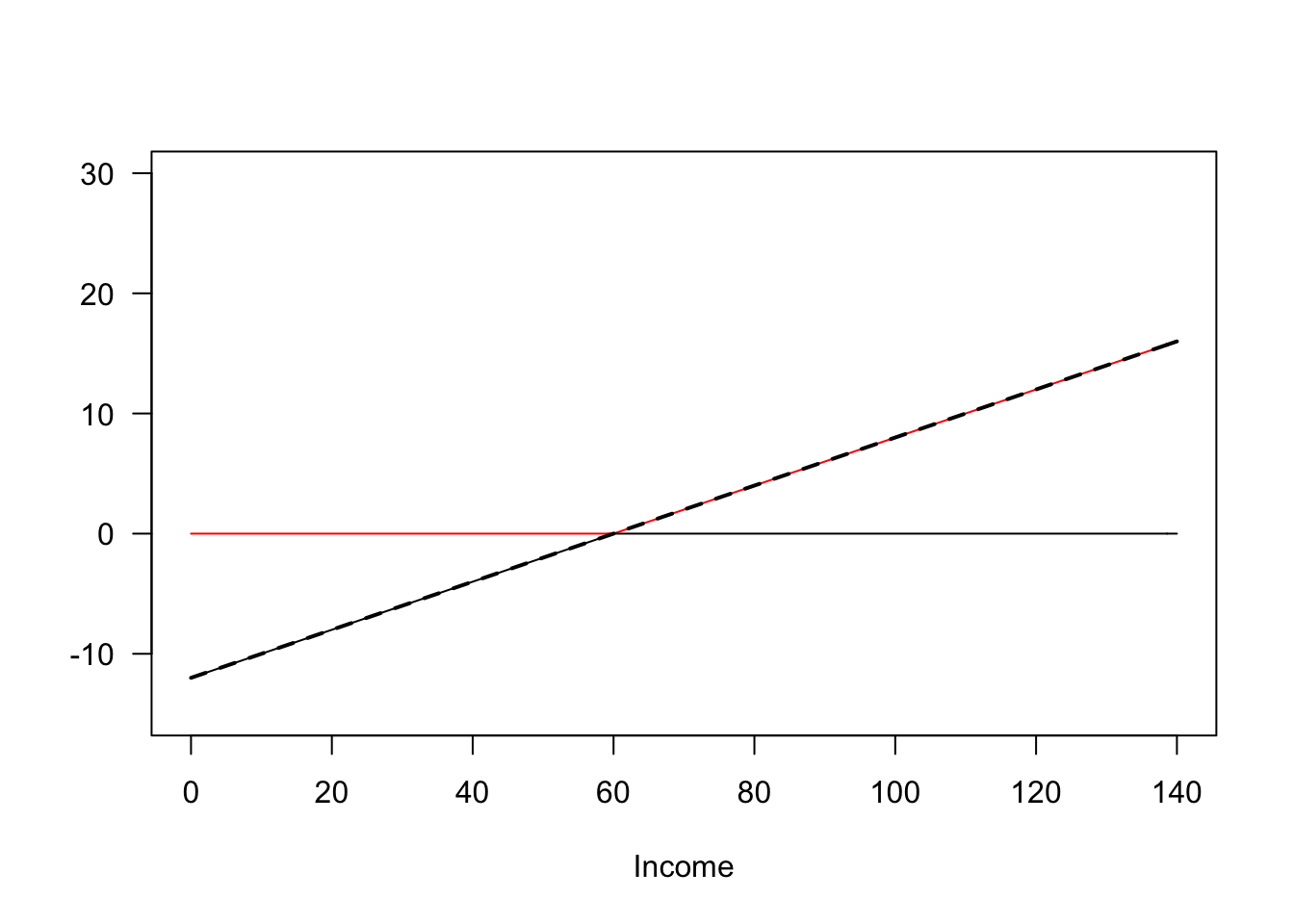# Should Government Programs be Means-Tested?

## 2020/01/07

Today, we’ll address a question that comes up frequently in policy discussions: whether government programs should be means-tested. For example, should a “basic income” program be available to all, or only those making below a certain income level? Similarly, one might ask whether it should be paid for by taxes on all, or only on the rich. This post raises a simple but important point, and is largely copied from Greg Mankiw. To make our question concrete, let’s compare the following basic income policies:

Policy A: a universal basic income of $1,000/month, paid for with a flat tax of 20% on income. Policy B: a means-tested transfer of$1,000 per month aimed at the truly needy. The full amount goes to those with zero income. The transfer is phased out: recipients lose 20 cents of it for every dollar of income they earn. These transfers are financed by a progressive income tax: The government taxes 20 percent on all income above \$60,000 per year

The latter policy seems much more progressive: it gives the largest transfer to those with the lowest incomes, and only taxes those with high incomes. In fact, the two policies are mathematically equivalent: the net transfer is identical for every income level! We can see this in Figure 1 (code included below). This shows that the discussion of who benefits and who pays for policies can be very misleading.

flat_tax = Vectorize(function(x){return(.2*x)})
universal_income = Vectorize(function(x){return(-12)})

progressive_tax = Vectorize(function(x){return(0.2*max(x-60,0))})
meanstested_income = Vectorize(function(x){return(-.2*max(60-x,0))})

plot(flat_tax,xlim=c(0,140),ylim=c(-15,30),las=1,ylab='',xlab='Income',xaxs='i',yaxs='i',col='red')

plot(progressive_tax,xlim=c(0,140),ylim=c(-15,30),las=1,ylab='',xlab='Income',col='red')
plot(function(x){progressive_tax(x)+meanstested_income(x)},xlim=c(0,140),add=TRUE,lwd=2,lty='dashed')